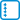## 2-factorization of 2k-regular graph

Prove Petersen’s theorem that every $$2k$$-regular graph can be decomposed into $$k$$ edge-disjoint 2-factors. Note that a $$k$$-factor is a graph in which all vertices have degree $$k$$.

• #### Hint

By using Eulerian trail, reduce the search of a 2-factor in a $$2k$$-regular graph to the search for a perfect matching in a $$k$$-regular bipartite graph.

• #### Solution

Without loss of generality, assume that the given graph $$G$$ is connected, because otherwise we decompose each component separately.

Because the given graph is $$2k$$-regular, it has all degrees even, and therefore it can be drawn in one closed trail. We orient the edges of the graph according to the trail. For each vertex, the indegree and outdegree are be exactly $$k$$.

Divide each vertex into two, keeping only the outgoing edges for one and only the incoming edges for the other. The result is a $$k$$-regular bipartite graph $$B$$. As a consequence of Hall’s theorem we may decompose the edges of any bipartite $$k$$-regular graph into $$k$$ perfect matchings.

The edges of each matching in $$B$$ form the asked 2-factor in the graph $$G$$, because each vertex of $$G$$ corresponds to two vertices in $$B$$. In addition, these vertices are not connected by an edge in $$B$$ (this would correspond to a loop in $$G$$) and both are incident with one matching edge – for each vertex of the pair the edge is different.